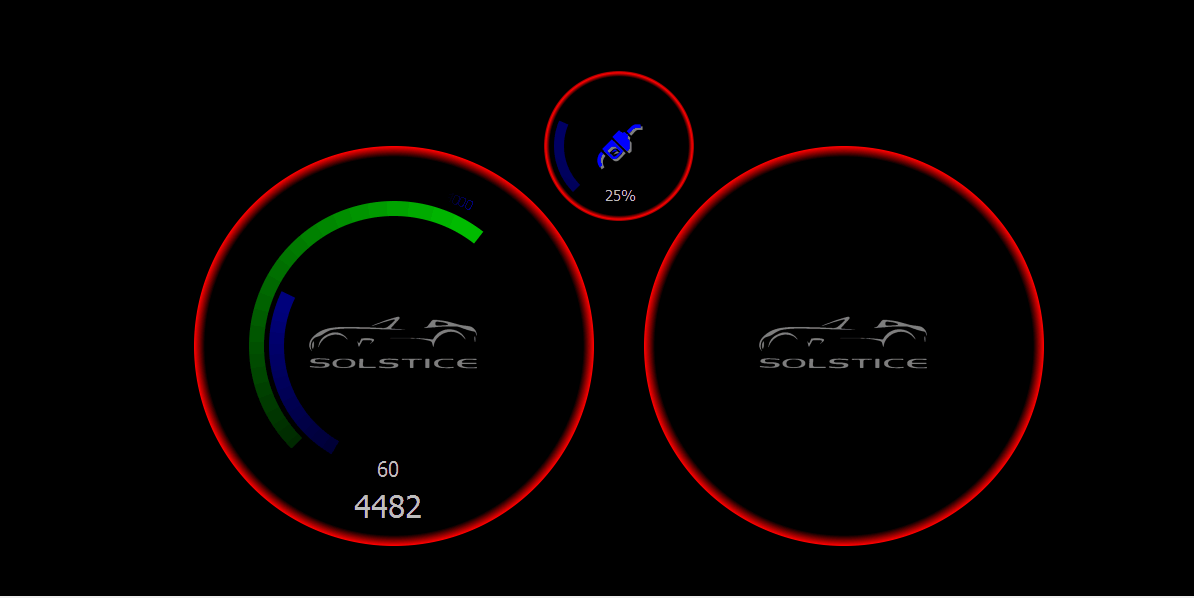# rendering a gradient using a mask

• What I am attempting to do is to draw onto a QBitmap some text that follows an arc. I am then creating a QConicalGradient that is transparent at either end of the gradient and in the middle of it is a color. when creating the gradient I set the rotation based on an input. This input is what is going to "point" the colored section of the gradient at a specific piece of text.

rendering the text onto the screen by setting the the pen to the gradient is difficult to do because the text is rotated to follow an arc. trying to map where the center of the conical gradient should be after using transform and rotate to render the text I have not been able to do.

So I opted to render the text to a QBitmap and then use that as a mask where I draw in the gradient. This does work but there is an anomaly, the text that should be completely transparent ends up being white. But not always.

Here is an animated GIF showing the problem.The code is rather large and I am not able to upload zip files or .py files. Here is the code for inside of the paintEvent

``````    def paintEvent(self, evt):
if None in (
self._speed_polygon,
self._rpm_polygon,
self._bezel_polygon,
):
return

bitmap = QBitmap (self.width, self.height)
bitmap.clear()
painter = QPainter(bitmap)
# painter.setRenderHint(QPainter.Antialiasing, False)
# painter.setPen(Qt.NoPen)
# painter.setBrush(QBrush(Qt.color0))
# painter.drawRect(0, 0, self.width, self.height)

font = painter.font()
font_size = font.pointSize()
font_size = int(round(font_size * 2.5))
font.setPointSize(font_size)
painter.setFont(font)

rpm = self._rpm / 7000

outer_radius = (min(self.width, self.height) / 2) * 0.775

painter.setBrush(Qt.NoBrush)
painter.setPen(QPen(Qt.color1, 6))

for i in range(8):
value1 = (i * 1000) / 7000
value2 = ((i + 1) * 1000) / 7000
value3 = ((i - 1) * 1000) / 7000

if value1 < rpm < value2:
diff = value2 - value1
value1_stop = value1 + (diff / 2)
if rpm <= value1_stop:
font_factor = _remap(rpm, value1_stop, value1, 0.5, 0.75)
else:
font_factor = 0.5
elif value1 > rpm > value3:
diff = value1 - value3
value1_start = value1 - (diff / 2)
if rpm >= value1_start:
font_factor = _remap(rpm, value1_start, value1, 0.5, 0.75)
else:
font_factor = 0.5
elif value1 == rpm:
font_factor = 0.75
else:
font_factor = 0.5

f = QFont(font)
f.setPointSize(int(round(font_size * font_factor)))
painter.setFont(f)

angle = _remap(i * 1000, 0.0, self._max_rpm, 0.0, 270.0)
text_angle = -(angle - 45) + 85

t = angle + 135.0
x = (outer_radius * math.cos(math.radians(t))) + (self.width / 2)
y = outer_radius * math.sin(math.radians(t)) + (self.height / 2)
point = QPointF(x, y)

painter.save()
painter.translate(point)
painter.rotate(-text_angle)
painter.drawText(0, 0, str(i * 1000))
painter.restore()

painter.end()
del painter

self._pixmap = bitmap

painter = QPainter(self)
painter.setRenderHint(QPainter.Antialiasing)

font = painter.font()
font.setPointSize(font.pointSize() * 2)
painter.setFont(font)
painter.setPen(QPen(QColor(255, 255, 255, int(round(190 * self._brightness)))))

speed = str(int(round(self._speed)))
if self._speed < 10:
painter.drawText(188, 310, 60, 40, 0, speed)
elif self._speed < 100:
painter.drawText(183, 310, 60, 40, 0, speed)
else:
painter.drawText(178, 310, 60, 40, 0, speed)

rpm = str(int(round(self._rpm)))
font.setPointSize(int(round(font.pointSize() * 1.5)))
painter.setFont(font)

if self._rpm < 10:
painter.drawText(170, 340, 70, 40, 0, rpm)
elif self._rpm < 100:
painter.drawText(165, 340, 70, 40, 0, rpm)
else:
painter.drawText(160, 340, 70, 40, 0, rpm)

painter.setPen(Qt.NoPen)

painter.setBrush(self._speed_gradient)
painter.drawPolygon(self._speed_polygon)

painter.setBrush(self._rpm_gradient)
painter.drawPolygon(self._rpm_polygon)
painter.setBrush(self._bezel_gradient)
painter.drawPolygon(self._bezel_polygon)

painter.setBrush(QColor(0, 0, 0, 0))
painter.setPen(Qt.NoPen)
painter.drawRect(0, 0, self.width, self.height)
painter.setBrush(self._rpm_number_gradient)
painter.setClipRegion(QRegion(self._pixmap))
painter.setCompositionMode(QPainter.CompositionMode_Clear)
painter.drawRect(0, 0, self.width, self.height)
painter.setCompositionMode(QPainter.CompositionMode_Source)
painter.drawRect(0, 0, self.width, self.height)

# painter.drawImage(QPointF(0, 0), self._pixmap)
event.set()
``````

and here is the code for the creation of the gradient use in the text

``````    def rpm(self, value):
angle = _remap(value, 0.0, self._max_rpm, 0.0, 270.0)
self._rpm_polygon = self._create_rpm_polygon(int(round(angle)))
self._rpm = value

x = self.width / 2.0
y = self.height / 2.0
self._rpm_number_gradient = QConicalGradient(QPointF(x, y), -(angle - 45))
self._rpm_number_gradient.setColorAt(0.45, QColor(0, 0, 0, 0))
self._rpm_number_gradient.setColorAt(0.5, QColor(0, 0, 255, int(round(255 * self._brightness))))
self._rpm_number_gradient.setColorAt(0.55, QColor(0, 0, 0, 0))

#
# self._rpm_number_gradient.setColorAt(rpm_end, QColor(0, 0, 0, 0))
# self._rpm_number_gradient.setColorAt(rpm_value, QColor(0, 0, 255, int(round(255 * self._brightness))))
# self._rpm_number_gradient.setColorAt(rpm_start, QColor(0, 0, 0, 0))
self.update()
``````

any help is greatly appreciated.

• I figured out what was wrong. I am used to other GUI frameworks where a call to update() is going to block until the update is done. This is not the case with Qt. By having the gradient created outside of the paint event the gradient can be in a state that is not fully populated with the needed stops when the gradient gets used to paint.

I need to figure out a way to get the code base to render faster. or figure out what is causing a variable delay between update() is called and when the paint event occurs. This can be a swing as large as 250 milliseconds which is not acceptable when trying to have real time animations based on an input.